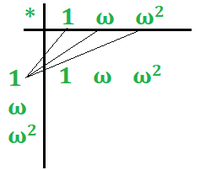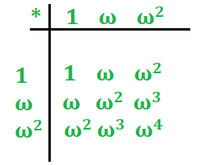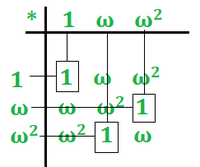# Solving Algebraic Structures Problems by Composition Table

• Difficulty Level : Expert
• Last Updated : 05 Mar, 2021

Problem-1:
Set G = { 1, ω, ω2 } i.e., three roots of unity and form a finite abelian group with respect to multiplication, also prove this statement by  composition table.

Explanation :
Given,  Set=G={1, ω, ω2 } , operation=‘*’  i.e. multiplication.

To prove that three roots of unity form a finite abelian group we must satisfy the following five properties that is Closure Property, Associative Property, Identity Property, Inverse Property, and Commutative Property.

Note-: ω3=1

1) Closure Property –

```∀ a , b ∈ G ⇒ a * b ∈ G
a=1 , b=ω ∈ G
⇒  1 * ( ω ) = ω = ω ∈ G```

Hence, Closure Property is satisfied.

2) Associative Property –

```(a* b) * c = a*(b *c)          ∀ a , b , c ∈ G
Let a=1, b=ω and c=ω2
So,
LHS  = ( a * b )*c
= (1*  ω ) *ω2 = ω3=1

RHS  = a * ( b * c)
= 1*( ω* ω2 ) = ω3= 1

Hence, RHS = LHS```

Associative Property is also Satisfied

3) Identity Property –

```a *e = a      ∀  a ∈ G
e=identity=1 (in case of multiplication)
1 ∈  G
Let a=1
1*1= 1
1 ∈  G
Identity property is also satisfied.```

4) Inverse Property –

Here we can see that inverse of 1 is 1, inverse of ω is ω2 and inverse of ω2 is ω . These inverse belong to set G.

So, Inverse property is also satisfied.

5) Commutative Property –

```a * b = b * a          ∀ a , b ∈ G
Let a=1, b=ω
LHS = a * b
= 1*ω = ω
RHS = b * a
= ω *1= ω
LSH=RHS```

Commutative Property is also satisfied.

We can see that all five property is satisfied. Hence, Three roots of unity form a finite abelian group with operation multiplication.

Forming Composition Table :

Step-1:
Write all elements of set in row and column and given operation ( * ) on the corner  and multiply the elements of the column with row element one by one and write it in the row as shown in the figure given below.Step-2:
After multiplying each element of column with row elements our composition table will look like figure given below,Step-3:
We know that,

`ω3=1 So, ω4=ω3.ω=1.ω=ω`

so our composition table becomesStep-4:
Finding inverse of elements.

Draw horizontal and vertical line from identity elements in each row, the vertical line provides the inverse of row elements, we can clearly see that inverse of 1 is 1, inverse of ω is ω2 and inverse of ω2 is ω.Step-5:
Satisfying Properties of Abelian Group from Composition Table

1. We see in the composition table all numbers are in set G, Hence Closure Property is satisfied.
2. We see that all numbers in composition table belong to set G, Hence Associative Property is satisfied.
3. In composition table in each row there is identity element 1, Identity Property is satisfied.
4. We see that inverse of 1 is 1, inverse of ω is ω2 and inverse of ω2 is ω. All belongs to set G, hence Inverse Property is also satisfied.
5. All numbers in composition table belongs to set G , Commutative Property is also satisfied.

Hence, G = { 1, ω, ω2 } is an abelian group with respect to multiplication.

Problem-2:
Set G = { 1, -1 , i , -i } i.e., four roots of unity and form a finite abelian group with respect to multiplication.

Explanation :
Four roots of unity are 1 ,-1 , i , -i . So our set will be G={ 1 , -1 , i , -i }

Operation = ‘*’ i.e. multiplication.

To prove that four roots of unity form a finite abelian group we must satisfy the following five properties that are Closure Property, Associative Property, Identity Property, Inverse Property, and Commutative Property.

1) Closure Property –

```∀ a , b ∈ G ⇒ a * b ∈ G
a=i , b= -i ∈ G
⇒  i * ( -i ) = -i2 = - ( -1 )
=1 ∈ G```

Hence, Closure Property is satisfied.

2) Associative Property –

```( a* b ) * c = a*( b *c)    ∀ a , b , c ∈ G
Let a=1, b=-1 and c=i
So, LHS= ( a * b )*c
= (1* ( -1 ) ) * i = -i
RHS= a * ( b * c)
=1*( -1* i ) = -i
Hence, RHS = LHS```

Associative Property is also Satisfied

3) Identity Property –

```a *e = a      ∀  a ∈ G
e=identity=1 (in case of multiplication)
1 ∈  G
1*1= 1
1 ∈  G```

Identity property is also satisfied.

4) Inverse Property –

`a * ( 1/a ) = 1       ∀  a ∈ G ,  1/a ∈ G  `

Here we can see that inverse of 1 is 1, inverse of -1 is 1, inverse of i is -i and inverse of -i is i. These inverse belong to set G.

So, Inverse property is also satisfied.

5) Commutative Property –

```a * b = b * a          ∀ a , b ∈ G
Let a=1, b=-1
LHS = a * b
= 1*( -1 ) = -1
RHS = b * a
= 1* ( -1 )= -1
LSH=RHS```

Commutative Property is also satisfied.

We can see that all five property is satisfied. Hence, Four roots of unity form a finite abelian group with operation multiplication.

My Personal Notes arrow_drop_up